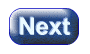created 08/26/97; revised 08/12/00; 07/23/03

# CHAPTER 8 — Length, Orthogonality, and the Column Matrix Dot Product

This chapter discusses two important facts: (1) The dot product of a column matrix with itself returns the square of the length of the vector it represents. (2) The dot product of two column matrices that represent orthogonal vectors is zero.

• The dot product and vector length.
• Unit vectors.
• Unit normal.
• Dot products of orthogonal vectors and of their column matrices.
• How to construct a vector orthogonal to a given vector.

### QUESTION 1:Let v = ( 3, 4 )T

1. v · v = ?
2. The length of v = ?